# Insert Character or Text to Cells

This post will guide you how to insert character or text in middle of cells in Excel. How do I add text string or character to each cell of a column or range with a formula in Excel. How to add text to the beginning of all selected cells in Excel. How to add character after the first character of cells in Excel.

Assuming that you have a list of data in range B1:B5 that contain string values and you want to add one character “E” after the first character of string in Cells. You can refer to the following two methods.

## Insert Character or Text to Cells with a Formula

To insert the character to cells in Excel, you can use a formula based on the LEFT function and the MID function. Like this:

`=LEFT(B1,1) & "E" & MID(B1,2,299)`

Type this formula into a blank cell, such as: Cell C1, and press Enter key. And then drag the AutoFill Handle down to other cells to apply this formula.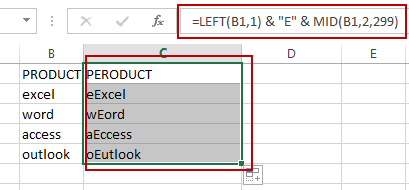This formula will inert the character “E” after the first character of string in Cells. And if you want to insert the character or text string after the second or third or N position of the string in Cells, you just need to replace the number 1 in Left function and the number 2 in MID function as 2 and 3. Like below:

`=LEFT(B1,2) & "E" & MID(B1,3,299)`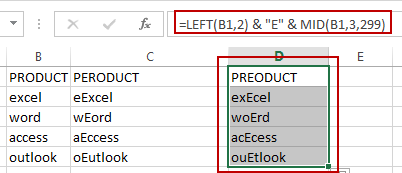## Insert Character or Text to Cells with VBA

You can also use an Excel VBA Macro to insert one character or text after the first position of the text string in Cells. Just do the following steps:

#1 open your excel workbook and then click on “Visual Basic” command under DEVELOPER Tab, or just press “ALT+F11” shortcut.#2 then the “Visual Basic Editor” window will appear.

#3 click “Insert” ->”Module” to create a new module.#4 paste the below VBA code into the code window. Then clicking “Save” button.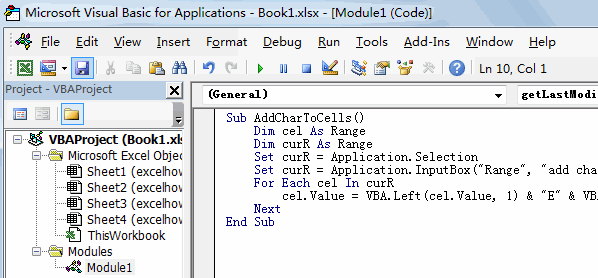```Sub AddCharToCells()
Dim cel As Range
Dim curR As Range
Set curR = Application.Selection
Set curR = Application.InputBox("select one Range that you want to insert one character", "add character to cells", curR.Address, Type: = 8)
For Each cel In curR
cel.Value = VBA.Left(cel.Value, 1) & "E" & VBA.Mid(cel.Value, 2, VBA.Len(cel.Value) - 1)
Next
End Sub```

#5 back to the current worksheet, then run the above excel macro. Click Run button.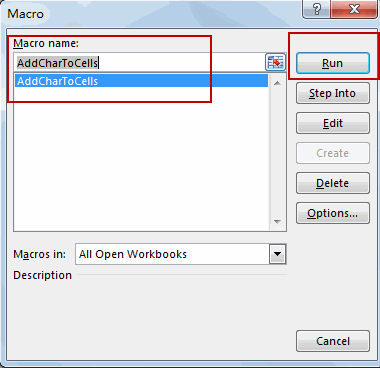#6 select one Range that you want to insert one character.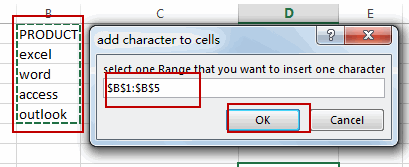#7 lets see the result.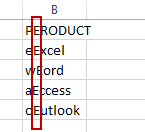### Related Functions

• Excel MID function
The Excel MID function returns a substring from a text string at the position that you specify.The syntax of the MID function is as below:= MID (text, start_num, num_chars)…
• Excel LEFT function
The Excel LEFT function returns a substring (a specified number of the characters) from a text string, starting from the leftmost character.The LEFT function is a build-in function in Microsoft Excel and it is categorized as a Text Function.The syntax of the LEFT function is as below:= LEFT(text,[num_chars])…

Related Posts

Find and Replace Multiple Values

This post will guide you how to find and replace multiple values at once with VBA macro or using formula  in Excel. How do I make multiple find and replace in Excel. Suppose that you have a few cells containing ...

VBA Macro For VLOOKUP From Another Sheet

In the previous post, you should know that how to fix or remove the #N/A error when using VLOOKUP formula to lookup value from another sheet. And this post will show you how to use VBA code to vlookup data ...

How To Insert Comments in Protected Worksheet in Excel

This post will show you how to allow comments in a protected worksheet in Excel. You can easily to insert comments into cells in a normal worksheet in Excel, but if want to insert a comment in a worksheet that ...

How To Convert Text to Upper Cases(Using VBA) in Excel

This post will show you how to switch from lower case to upper case in Excel. and I am going to show you two different ways of converting text to upper cases using formula or VBA macro in Excel 2013,Excel ...

How To Hide Every Other Row in Excel (Using VBA)

This post will show you how to hide alternate rows or columns in Excel or how to hide every third, fourth, fifth row or column in Excel. If you want to hide every other row in your current worksheet, how ...

Sidebar